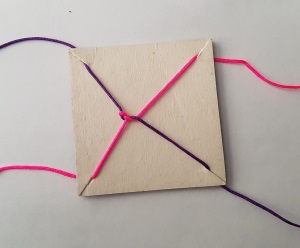#### You may also like### Tweedle Dum and Tweedle Dee

Two brothers were left some money, amounting to an exact number of pounds, to divide between them. DEE undertook the division. "But your heap is larger than mine!" cried DUM...### Sum Equals Product

The sum of the numbers 4 and 1 [1/3] is the same as the product of 4 and 1 [1/3]; that is to say 4 + 1 [1/3] = 4 ï¿½ 1 [1/3]. What other numbers have the sum equal to the product and can this be so for any whole numbers?### Special Sums and Products

Find some examples of pairs of numbers such that their sum is a factor of their product. eg. 4 + 12 = 16 and 4 × 12 = 48 and 16 is a factor of 48.

# Twisting and Turning

##### Age 11 to 14Challenge Level

Twisting has the effect of adding 1: $$x\mapsto x + 1$$ Turning transforms any number into the negative of its reciprocal: $$x\mapsto -\frac{1}{x}$$ Starting at zero, these five moves: Twist, twist, twist, turn, twist
produce:$$0, 1, 2, 3, -\frac{1}{3}, \frac{2}{3}$$

Can you continue from there and then return to zero? You might find it helpful to record each step in a table.

Take another look at the video.
The team use a strategy to help them get back to zero.
Can you figure out how they decide when to stop twisting and start turning?

If you want to have a go at the trick for yourself, but don't have enough people or skipping ropes, you can also perform the tangling and untangling process using a small piece of card and two pieces of string.